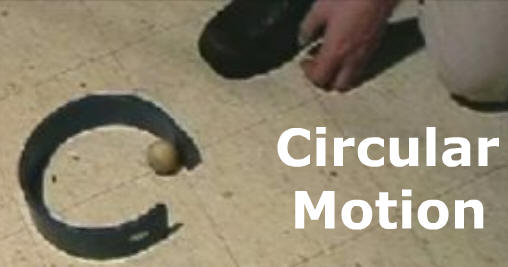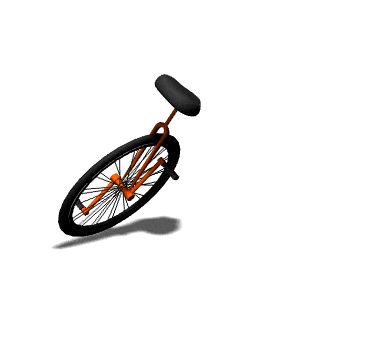Review - Velocity, Circular Motion[ Flash ] [ HTML5 ] Rutgers U. Videos

A. Equations & VectorsReview

Why is an object moving at constant speed
in a circle considered to be accelerating?Because while its speed is not changing,
its direction is.

1. Centripetal Acceleration (ac) - acceleration toward center of curvature

 a)Equation: ac = V2 r

V - velocity

b) (V) Instantaneous Velocity - tangent to circle, in the direction of the motion

c) Centripetal Force (Fc) - force directed toward the center of the circleEx) A car moving in a circular path with a radius of 2.0 m has a velocity of 8.0 m/s.   What is the centripetal acceleration of this car?

Ex) A car moving in a circular path with a radius of 2.0 m has a velocity of 8.0 m/s. What is the centripetal acceleration of this car?

 ac = V2 r

 ac = (8.0 m/s)2 2.0 m

ac = 64/2

ac = 32. m/s2

Circular Velocity Game (Flash)

[ HTML5 ] GameGiancoli p. 130) 2, 4